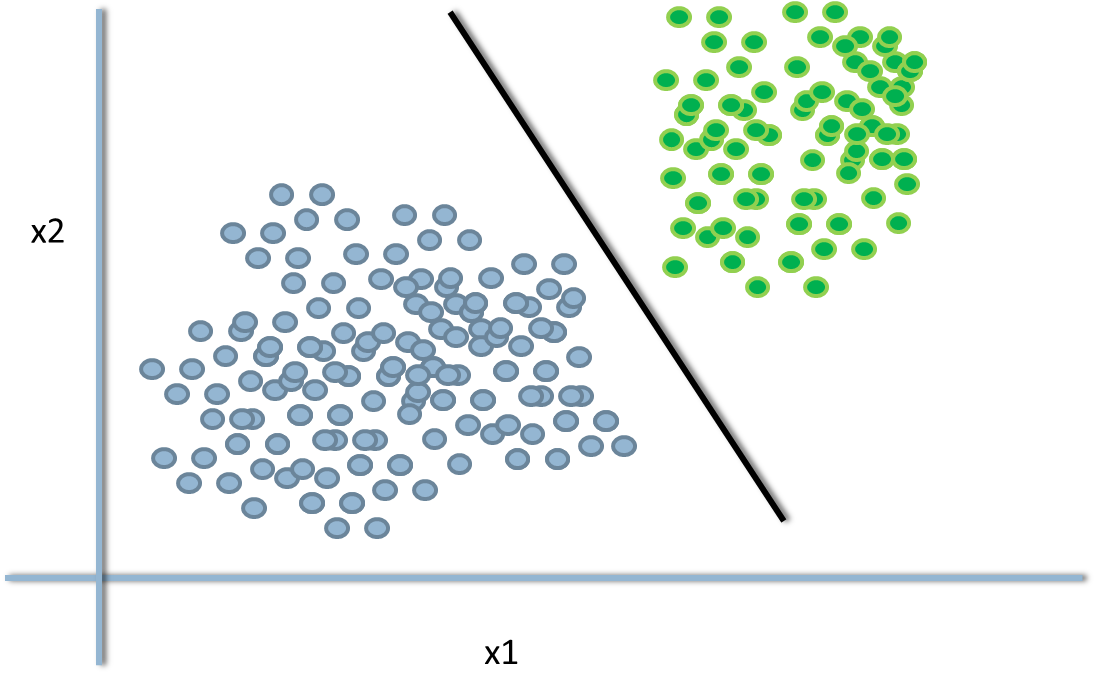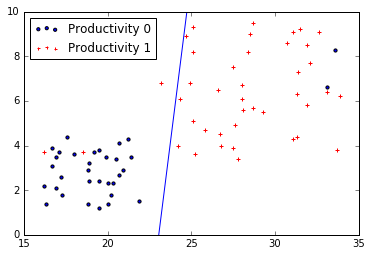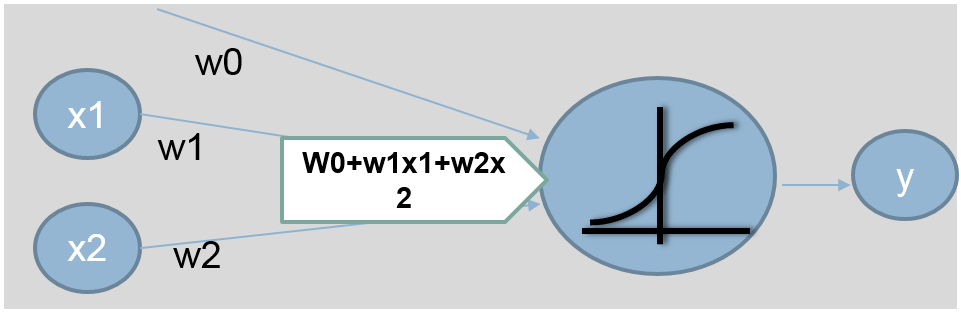• No products in the cart.

# 204.5.2 Decision Boundary – Logistic Regression

##### Few more things before getting into Neural Networks.

Link to the previous post : https://statinfer.com/204-5-1-neural-networks-a-recap-of-logistic-regression/

In the last session we recapped logistic regression. There is something more to understand before we move further which is a Decision Boundary. Once we get decision boundary right we can move further to Neural networks.

### Decision Boundary – Logistic Regression

• The line or margin that separates the classes.
• Classification, algorithms are all about finding the decision boundaries.
• It need not be straight line always.
• The final function of our decision boundary looks like,
• Y=1 if $$w^Tx+w_0>0$$ ; else Y=0• In logistic regression, it can be derived from the logistic regression coefficients and the threshold.
• Imagine the logistic regression line p(y)=$$e^(b_0+b_1x_1+b_2x_2)/1+exp^(b_0+b_1x_1+b_2x_2)$$
• Suppose if p(y)>0.5 then class-1 or else class-0
• $$log(y/1-y)=b_0+b_1x_1+b_2x_2$$
• $$Log(0.5/0.5)=b_0+b_1x_1+b_2x_2$$
• $$0=b_0+b_1x_1+b_2x_2$$
• $$b_0+b_1x_1+b_2x_2=0 is the line$$
• Rewriting it in mx+c form
• $$X_2=(-b_1/b_2)X_1+(-b_0/b_2)$$
• Anything above this line is class-1, below this line is class-0
• $$X_2>(-b_1/b_2)X_1+(-b_0/b_2)$$ is class-1
• $$X_2<(-b_1/b_2)X_1+(-b_0/b_2)$$ is class-0
• $$X_2=(-b_1/b_2)X_1+(-b_0/b_2)$$ tie probability of 0.5
• We can change the decision boundary by changing the threshold value(here 0.5)

### Practice : Decision Boundary

• Draw a scatter plot that shows Age on X axis and Experience on Y-axis. Try, to distinguish the two classes with colors or shapes (visualizing the classes)
• Build a logistic regression model to predict Productivity using age and experience.
• Finally, draw the decision boundary for this logistic regression model.
• Create, the confusion matrix.
• Calculate, the accuracy and error rates.

Solution : We have covered all these tasks in previous post. However, we will again plot the decision boundary.

In :
import matplotlib.pyplot as plt

fig = plt.figure()

ax1.scatter(Emp_Productivity1.Age[Emp_Productivity1.Productivity==0],Emp_Productivity1.Experience[Emp_Productivity1.Productivity==0], s=10, c='b', marker="o", label='Productivity 0')
ax1.scatter(Emp_Productivity1.Age[Emp_Productivity1.Productivity==1],Emp_Productivity1.Experience[Emp_Productivity1.Productivity==1], s=10, c='r', marker="+", label='Productivity 1')
plt.legend(loc='upper left');

x_min, x_max = ax1.get_xlim()
ax1.plot([0, x_max], [intercept1, x_max*slope1+intercept1])
ax1.set_xlim([15,35])
ax1.set_ylim([0,10])
plt.show()We did cover this part in our last post too, but we will move further for one or two more posts to understand different kind of decision boundaries.

### New Representation for Logistic Regression

y=e(b0+b1x1+b2x2)1+e(b0+b1x1+b2x2)

y=11+e(b0+b1x1+b2x2)

y=g(w0+w1x1+w2x2)whereg(x)=11+e(x)

y=g(wkxk)#### Finding the weights in logistic regression

out(x) = y=g(wkxk)The above output is a non linear function of linear combination of inputs – A typical multiple logistic regression line

We find w to minimize ni=1[yig(wkxk)]2

The next post is a practice session on non-linear decision boundary.

Link to the next post : https://statinfer.com/204-5-3-practice-non-linear-decision-boundary/

0 responses on "204.5.2 Decision Boundary - Logistic Regression"